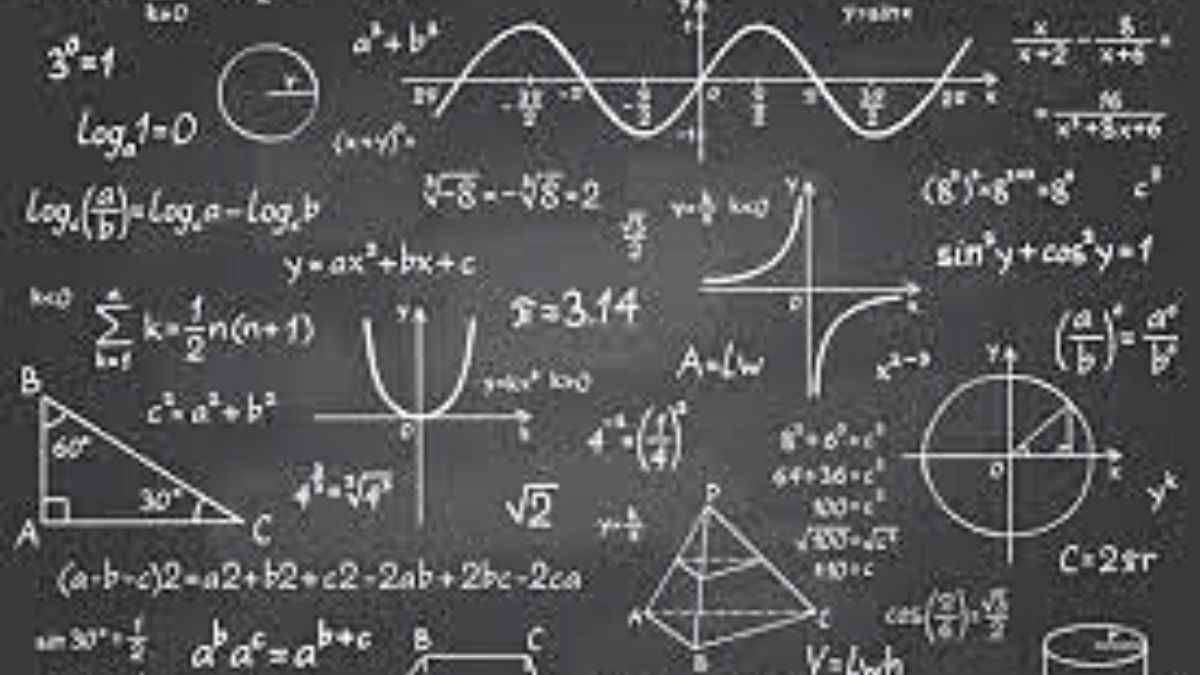# Another set of interesting math riddles for you!

Math is fun. Don’t believe us? Try these fun-filled math riddles.Welcome back to the fun-filled part of the day mathematicians. We have some awesome riddles that will blow your mind today. I bet you cannot solve all of them. Want to give it a shot? Let’s start then -

MATH RIDDLE 1

The ages of a father and son add up to 66. The father's age is the son's age reversed. How old could they be? (3 possible solutions).

MATH RIDDLE 2

What two whole, positive numbers have a one-digit answer when multiplied and a two-digit answer when added?

MATH RIDDLE 3

You want to boil a two-minute egg. If you only have a three-minute timer (hourglass), a four-minute timer, and a five-minute timer, how can you boil the egg for only two minutes?

MATH RIDDLE 4

How much will a 38° angle measure when looked at under a microscope that magnifies ten times?

MATH RIDDLE 5

1=5, 2=15, 3=215, 4=3215, 5=?

MATH RIDDLE 6

How can you make the following equation true by drawing only one straight line: 5+5+5=550? Can you figure it out?

MATH RIDDLE 7

In a certain city, 5% of all the people in town have unlisted phone numbers. If you select 100 names at random from that city's phone directory, how many people selected will have unlisted phone numbers?

Answer to riddle 1: 51 and 15. 42 and 24. 60 and 06.

Answer to riddle 2: 1 and 9.

Answer to riddle 3: Once the water is boiling, turn the three-minute timer and five-minute timer over. When the three-minute timer runs out, put the egg in the boiling water. When the five-minute timer runs out, two minutes have elapsed and it is time to take the egg out of the water. You don't need the four-minute timer for this riddle.

Answer to riddle 4: It will still be 38°

Answer to riddle 5: 5=1, because 1=5

Answer to riddle 6: Draw a line on the first plus sign that turns it into a 4! The equation then becomes true: 545+5=550. You could also change the equal symbol to a crossed-out equal symbol which means "not equal to".

Answer to riddle 7: None. If their names are in the phone directory, they do not have unlisted phone numbers!

Were you able to get the answers for all of them? If so, great!! For those who couldn’t, better luck next time. Till then, Doodles!!!

Get the latest General Knowledge and Current Affairs from all over India and world for all competitive exams.
खेलें हर किस्म के रोमांच से भरपूर गेम्स सिर्फ़ जागरण प्ले पर